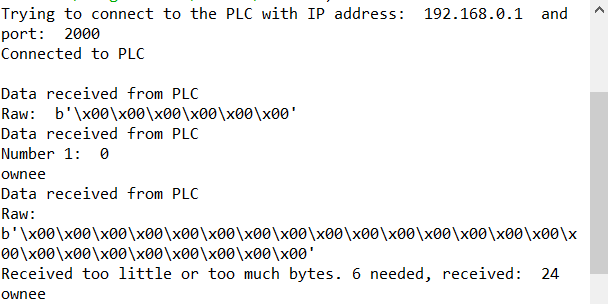# Communication between Python and PLC through TCP

@4lloyd, a question:

I’ve got the following PLC programme:

Which is communicating with this Python code:

``````def check_transport_band(conn):
number1 = 0
try:
data = conn.recv(512)
print("Raw: ", data)
except Exception as e:
print("Error while receiving data. Error message: ", str(e))
return None

if not data: return None

if (len(data) == 6):
number1 = int(struct.unpack(">h", data[0:2]))
print("Number 1: ", number1)
else:
print("Received too little or too much bytes. 6 needed, received: ", len(data))
if number1== 1:
# or number2 or number3
print ("owkee")
return True

else:
print ("ownee")
return False
``````

Now, the first time start the programme, when the starting signal is (0/ band off), I receive data which has a length of 6 bits (as expected).

However, the second time around I receive 24 bits:When start the programme, when the starting signal is (1/ band on), I receive data which has a length of 6 bits (as expected).

However, when i turn it of it starts to range between 30 and 24.

Would you have any idea what’s going on here?

I think that the problem is that the PLC is sending data more often then your script is checking for it. Therefor there are more than 6 bytes in the receive buffer.

``````data = data[-6:]
``````if not data: return None